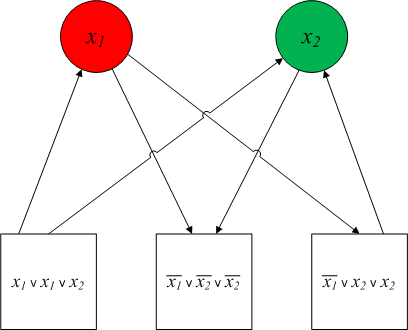# Funny graph coloring redux

So, that proof I posted on Monday kind of sucked! Here's my second attempt.

Again, the problem:

Let a "funny graph" be a finite directed-graph where each vertex is either "square" or "circular" (but not both), and each edge is between a square vertex and a circular vertex (in either order). Prove that the following problem is NP-complete.

• Given a funny graph, is there a way to color the circular vertices red or green, so that every square vertex either has an out-edge to a green vertex, or an in-edge from a red vertex.

And here's the new proof:

Let FGCOLOR = {⟨G⟩| G is a funny graph that is colorable so that every square vertex either has an out-edge to a green vertex or an in-edge from a red vertex}.

To show that FGCOLOR is NP-complete, first observe that FGCOLOR is in NP, since, given a graph G and a subset S of G's nodes (the nodes marked as "square"), it would take linear time in the size of S to examine each node of S and verify that it meets the conditions of FGCOLOR.

Since FGCOLOR is in NP, we can show that FGCOLOR is NP-complete by giving a polynomial time reduction from 3SAT. In this reduction, we make use of the correspondence between circular nodes in a graph which must be colored either green or red, and variables in a formula which must be assigned either true or false.

The reduction maps a 3cnf Boolean formula φ to a graph G. Construct G as follows:

• For each variable in φ, add a circular vertex to G and label it with that variable's name.
• For each clause in φ, add a square vertex to G. Then, for each square vertex, add edges as follows:
• If the corresponding clause in φ contains a literal that matches the label on a circular vertex, add an out-edge from the square vertex to the circular vertex.
• If the corresponding clause in φ contains a literal that contradicts the label on a circular vertex, add an in-edge to the square vertex from the circular vertex.

The following figure illustrates this construction when &phi = (x1x1x2) ∧ (¬x1 ∨ ¬x2 ∨ ¬x2) ∧ (¬x1x2x2). (The red and green coloring of the circular vertices isn't part of the construction, but it illustrates a later point.)Next, we show that φ is satisfiable if and only if ⟨G⟩ is in FGCOLOR. For the forward direction, suppose φ has a satisfying assignment. Color the circular vertices in G as follows: green if the corresponding variable in φ has been assigned true, and red if the corresponding variable has been assigned false. Since each clause in φ must evaluate to true, at least one literal in every clause must evaluate to true. That is, each clause must have at least one literal that either matches a variable that has been assigned true, or that contradicts a variable that has been assigned false. Therefore the square vertices representing each clause must have at least one of either an out-edge to a green vertex, or an in-edge to a red vertex. Therefore ⟨G⟩ is in FGCOLOR.

For the opposite direction, suppose ⟨G⟩ is in FGCOLOR. Then each circular vertex must be either red or green. Assign the variables in φ as follows: true if the corresponding circular vertex is green, and false if the corresponding circular vertex is red. Since each square vertex in G must have at least one of either an out-edge to a green vertex or an in-edge to a red vertex, each clause in φ must have at least one true literal. Therefore φ has a satisfying assignment.

We have shown that FGCOLOR is reducible from 3SAT in polynomial time. Since 3SAT is NP-complete and FGCOLORis in NP, FGCOLOR is NP-complete.

The key idea of the reduction is that circular vertices have to be either green or red -- not both, and not neither -- and that Boolean variables have to be either true or false -- not both, and not neither. Once I finally noticed that, I could exploit the correspondence, letting green be true and red be false.

Actually, I just realized that a thoughtful anonymous commenter with a ucalgary.ca IP address posted a hint to that effect this morning (not to mention their own version of the proof, in ROT13). Thanks!

• #### Join us at the Programming Languages Mentoring Workshop at ICFP next month

New blog post, in which it is time for my annual exhortation. This entry was originally posted at…

• #### How to create an .srt caption file for a video

New blog post, in which I serve in my capacity as ICFP accessibility co-chair, or something. This entry was originally posted at…

New blog post, in which I dispense advice. This entry was originally posted at https://lindseykuper.dreamwidth.org/35335.html. Please comment…

• Post a new comment

#### Error

Anonymous comments are disabled in this journal

default userpic